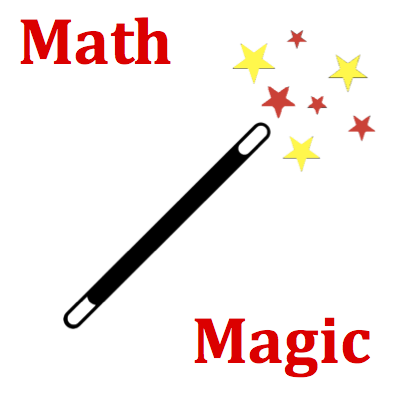# Vectors and Projectiles - Mission VP4 Detailed HelpIn the Vector Addition Lab, the following data was collected for determining the displacement from the door of the Physics classroom to another location in the building: 2 m, West; 14 m, South; 22 m, East; 19 m, North; and 2 m, West. The magnitude of the resultant displacement from the physics classroom to the assigned location is closest to ... .    (Note: Numbers are randomized numbers and likely different from the numbers listed here.)An effective strategy for all questions in this mission will center around a rough sketch of the addition of two vectors (See Think About It section). Consider the following steps:   Begin by simplifying the collection of small displacements by adding all the east-west vectors together. Consider west to be the negative direction and add the negative westward displacements to the positive eastward displacements. Record the result and label as ∑E-W (for sum of the east and west vectors). Repeat the process of adding the north-south vectors together, considering south to be the negative direction. Record the result and label as  ∑N-S (for sum of the north and south vectors). The two vector sums will now be added together. Consider the sum of the east-west vectors (∑E-W) as a single vector and sketch it in the appropriate direction. Place an arrowhead at the end of the vector. Consider the sum of the north-south vectors (∑N-S) as a single vector, Starting at the arrowhead of the first vector, draw this second vector in the appropriate direction and to the approximate length. Put an arrowhead at the end of the vector. Draw the resultant vector from the tail of the first to the arrowhead of the second vector. Label the vector as R (for resultant) and put an arrowhead at the end of the resultant vector. Since the resultant is a hypotenuse of a right triangle, the Pythagorean theorem can be used to calculate its magnitude (see Math Magic section).The head-to-tail method of vector addition should be used to create a rough sketch of this physical situation. In this method, the first vector (the ∑E-W vectors) is sketched (not to scale) in its indicated direction. The second vector (the ∑N-S vectors) is then sketched (not to scale) starting at the head (arrowhead) of the first vector. The resultant vector is then drawn from the tail of the first vector to the head of the last vector. The Pythagorean Theorem can then be used to calculate the magnitude of the resultant (see Math Magic section).Pythagorean Theorem When two vectors that make a right angle to each other are added together, the resultant vector is the hypotenuse of a right triangle. The Pythagorean theorem can be used to calculate the magnitude of the resultant. If the right triangle has sides with lengths of x and y, then the length of the hypotenuse is the square root of the sum of the squares of the sides. That is,   hypotenuse = SQRT (x2+ y2)